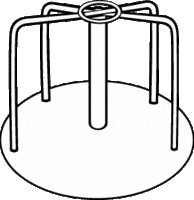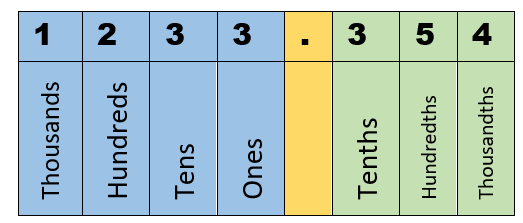# Math Worksheets Land

Math Worksheets For All Ages

# Math Worksheets Land

Math Worksheets For All Ages

Home > Math Topics > Rounding >

# Rounding Integers Worksheets

You find yourself in many different situations when it comes to math. In some cases, you will need a clear and accurate solution that is exact. In many other cases, you will simply need to have an idea of where the solution may reside in order to make a good choice. For instance if you were tasked with brining in napkins for a class lunch and the napkins you were buying came in packs of 10, you do not need calculate the exact number of students you were bringing napkins in for, you would just need to make sure you had a satisfactory number of napkins. We will explore how to round integers in both whole number and decimal forms. These worksheets and lessons reinforce the concept of place value and we have students learn how to round to fixed places.

### Aligned Standard: 4.NBT.3

• Answer Keys - These are for all the unlocked materials above.

### Homework Sheets

1. Find the underlined place value. 2. Complete the expanded form for each number in standard form. 3. Compare the place values.

• Place Homework 1 - Multiples of 100 always have zeroes in the ones and tens place.
• Expanded Homework 2 - If the hundred place number is 5 or higher, we round to the higher number.
• Compare Homework 3 - Multiples of 1000 always have zeroes in the ones, tens, and hundreds place.

### Practice Worksheets

I receive a great deal of positive feedback on these sheets. Most teachers love the diverse questioning technique.

• Practice 1 - If the hundred place number is 4 or less, we round down.
• Practice 2 - Determine the place value of the underlined number.
• Practice 3 - The best thing to do is to break the numbers into all the place values.

### Math Skill Quizzes

You will find that these are all relatively at the same level for the same skills.

• Quiz 1 - Round 4654 to the nearest tens place.
• Quiz 2 - I would start by underlining the place of interest.
• Quiz 3 - The best thing to do is to break the numbers down in place value chart and look at the digit that is significant. We highlight the significant digit in bold.

### How to Round Values Based on Place ValueSometimes you need rounding off of numbers to make them simpler and easier to understand. We often do this when we are looking to make a decision that we do not need to be exact with, but we just need a general idea or understanding of where it fits in order to make a well-informed decision. We come across these types of situations all the time. If ever had a birthday party with cupcakes you always have to estimate how many guests, there will be in order to prepare the correct amount of food. Rounding off is also used for eliminating the decimal points in the number.

Each digit in a number has a position called its place value. Look at the image below. To the left of the decimal point are integers, and we reference these place values as ones, tens, hundreds, thousands, and so on. To the right of the decimal point are decimals, referenced as tenths, hundredths, thousandths, etc. As we move from right to left in a number, each place value is ten times larger than the place value to its right. The opposite is true when you go in the opposite direction.There are a number of different reasons why in some circumstances it is more appropriate to round to a particular place value than others. You will need to contemplate this in each individual case. Once you select the proper place value to round to it is helpful to circle or underline that particular digit. Here is quick run down of how to work with these particular values.

Highlight the Digit of Interest - You will keep that number the same, for now, but move down to the next lower place value. This means that you will look to the digit that is located one place to the right. Is It 5 or Higher? If that value is 5 or higher you round the next place value up and make all the digits from the place value you rounded from 0 and all consecutive place values after. Is It Less Than 5? - If that value is a 4 or less, you will round the place above it down one integer. Once again all of the proceeding place values from where you considered rounding on are transformed to 0s.

Unlock all the answers, worksheets, homework, tests and more!
Save Tons of Time! Make My Life Easier Now

## Thanks and Don't Forget To Tell Your Friends!

I would appreciate everyone letting me know if you find any errors. I'm getting a little older these days and my eyes are going. Please contact me, to let me know. I'll fix it ASAP.